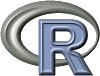Introduction
External Data Entry
Examples

## Introduction

This server allows the use of two packages developped in our Lab for the R software: ade4 and seqinr.

The documentation of the ade4 package is available here, and the documentation of the seqinr package is available here. Note that on this server, these two packages are automatically loaded each time R is launched, so you do not need to use the library(ade4) and library(seqinr) commands (but using them will not hurt).

To run Rweb just type the code you want to execute into the text window below and then click on the submit button. You will get a new html page with the text output of your code followed by the graphical output (if any) from your code. A detailed example of use is here. It shows how you can use this system to search sequence data banks for gene sequences, compute the codon frequences for these genes, and perform a correspondence analysis of this data table.

You can try examples from the ade4 package by just clicking the Submit button with the examples below. Just remove these lines to type your own code. The computer time for all of this is donated by the PBIL. Please note that all actions are logged and that abuse will lead to exclusion of IP addresses.

## External Data Entry

There are three ways to enter data for use in your Rweb session :
1- a text area where you can paste a dataframe.
2- a text area where you can enter the URL for a Web accessible dataset
3- a browse button for selecting a dataset on your computer
Of course, you should use only one of these three ways.

Either way, the dataset will be read in using read.table with header=T and stored in a dataframe called X. The dataframe, X, will then be attached so you can use the variable names. Temporary files are stored here. Eventually I hope to add several other options for data entry ... let me know if you have any suggestions.

1- Paste a dataframe here :

or

2- Enter a dataset URL :

or

3- Select a local file to submit:

## Examples

Here are a few examples of use of Rweb. You can copy the following code examples and paste them into the text window at the top of this page. Alternatively, if you are in a more contemplative mood, you can go to this page and just click and watch.

### Example 1

First example using the ade4 library, showing how to do a coinertia analysis :
```data(doubs)               # load the doubs data set
dudi1 <- dudi.pca(doubs\$env, scale = TRUE, scan = FALSE, nf = 3)    # first PCA
dudi2 <- dudi.pca(doubs\$fish, scale = FALSE, scan = FALSE, nf = 2)   # second PCA
coin1 <- coinertia(dudi1,dudi2, scan = FALSE, nf = 2)               # coinertia analysis
coin1                     # display coinertia analysis results
plot(coin1)               # plot coinertia results
r1 <- randtest(coin1)     # perform the permutation test
r1                        # display it
plot(r1)                  # plot it
```

### Example 2

Here is an example showing how you can search sequence data banks for gene sequences (with the seqinR package), compute the codon frequencies for these genes, and perform a correspondence analysis (CA) of the frequencies table. This example makes the CA of the codon frequencies in the coding sequences of Mycoplasma genitalium. The web page explaining this example is here.
```choosebank(bank = "emglib")                                  # chooose the emglib sequence bank
req <- query("liste", "sp=mycoplasma genitalium et t=cds")   # search the bank for sequences
seqs <- lapply(liste\$req[], getSequence)                     # retrieve the sequences
tabco <- lapply(seqs, uco)                                   # compute codon frequences
tabco <- as.data.frame(lapply(tabco, as.vector), row.names = names(tabco[]))   # make the dataframe
names(tabco) <- liste\$req[]                                  # setup codon names
ca <- dudi.coa(tabco, scan = F, nf = 3)                      # perform CA
s.label(ca\$co, clabel = 0, sub = "Genes F1xF2 map")          # plot Genes map
s.label(ca\$li, sub = "Codons F1xF2 map")                     # plot codons map
```

### Example 3

Here is another example showing how you can do a Principal COordinates analysis (PCO) on the distance matrix computed on a set of aligned sequences in Clustal format. This example also makes use of the seqinR package (for the dist.alignment function). The web page explaining this example is here.
```mat <- dist.alignment(aln, matrix = "similarity")
dst <- lingoes(mat)
cat <- as.factor(c(1,1,1,1,2,3,3,3,3,3,3,3,4,1,1,1,1,1,1,1,1,3,3,3,3,5,5,5,3))
pco <- dudi.pco(dst, scan = F, nf = 3)
s.label(pco\$li, sub = "F1xF2 map")
```

### Example 4

Here is a big example, which is in fact the reproduction of the figure from the paper by Charif, D., Thioulouse, J. Lobry, J.R., Perriere, G. in Bioinformatics (see here). This example makes intensive use of the seqinR package. The web page presenting this example is here.

### Example 5

Here is a code example showing how an external data set can be used. You must use one of the three data entry methods to provide data table X, and the PCA of this table will be computed.
```X                         # check that the data table has been read correctly
pca1=dudi.pca(X,scan=F)   # do the PCA of this table
scatter(pca1)             # PCA biplot
s.corcircle(pca1\$co)      # PCA correlarion circle
s.label(pca1\$li)          # PCA row scores
score(pca1)               # PCA canonical display : variables = f(principal components)
```

### Example 6

If you don't know how to program in R, here is a short code snippet that shows you a little bit about what R can do.
```x <- rnorm(100)           # 100 random numbers from a normal(0,1) distribution
y <- exp(x) + rnorm(100)  # an exponential function with error
result <- lsfit(x,y)      # regress x on y and store the results
ls.print(result)          # print the regression results
plot(x,y)                 # pretty obvious what this does
abline(result)            # add the regression line to the plot
lines(lowess(x,y), col=2) # add a nonparametric regression line (a smoother)
hist(result\$residuals)    # histogram of the residuals from the regression
```

© 2004 Jean Thioulouse - Based on the Rweb system by Jeff Banfield.